# The No-Three-In-Line Problem

### A Puzzle

Mark six more points (making twelve in all) without allowing three along any straight line.

The figure to the right shows a 6×6 grid with six of the points marked as clues. You can mark (or unmark) additional grid points by clicking on them. The problem:

• Mark six more points, making a total of 12.
• You can't have three marked points along any straight line.
• That includes not just horizontal and vertical lines, but lines of any slope.

Give it a try! You can reason the solution out logically from the given clues.

### A Family of Problems

"The No-Three-In-Line Problem" is really a family of problems centered around the general question, "How many markers can be placed on an n×n grid with no three in a line?" Some basic observations:

1. You can't have more than 2 in any row, so the answer can't be more than 2n.
2. To get 2n markers on an n×n grid, you need 2 in each row (and 2 in each column).

For small values of n (that is, small grid sizes), it's not hard to find solutions that place 2n markers with no three in a line, even working by hand. We'll look at some of these small grids as puzzles in the next few pages.

For larger values of n, it is not known whether 2n markers can be placed, or whether the maximum number is smaller than 2n. This is one of the outstanding questions.

### The 4×4 Solutions

Find all four ways to solve the 4×4 grid.
The solutions display a variety of symmetry types.

In all of the following pages, when I refer to a “solution” on the n×n grid, I mean an arrangement of 2n markers with no three in line.

There are essentially four different solutions on the 4×4 grid. Each of the diagrams to the right gives enough clues to determine one of the four solutions uniquely.

You can get other solutions by rotating or reflecting these four. If you take all of those different orientations into account then there are 11 distinct solutions in all.

It seems fair to assume that many mathematicians and puzzle enthusiasts have worked out the different solutions for small grids over the years. Beyond a certain point, enumerating the possibilities by hand becomes impractical, and computer searches have taken over.

The number of distinct solutions for each grid size up to 18×18 is known. These numbers are available in the Online Encyclopedia of Integer Sequences (Sequence A000769).

Although it is dated, the most comprehensive single site for information about this problem is still Achim Flammenkamp's No-Three-In-Line page. Among other things, you can find a table listing the number of solutions for different grid sizes, and you can download a file containing all the solutions known to Flammenkamp. That includes all of the solutions on grids up to 16×16, and certain solutions with larger sizes. Information about the complete set of solutions for n=17 and n=18 doesn't appear to have been published, other than the entry in the OEIS. If further information was once available on the web, it doesn't appear to be any longer.

### The 5x5 Solutions, Part 1

Find three solutions on the 5×5 grid (with generous clues).

On the 5×5 grid, there are five essentially different solutions. Clues for three of the solutions are shown above, and they should be easy to solve. The other two are on the next page. They should be a little more difficult.

#### Counting slopes

The 5×5 grid is the smallest where lines other than horizontal, vertical, and diagonal (slope ±1) are a factor. As the grid size increases, the number of different slopes to consider increases as well. There's a formula for the number of different slopes determined by pairs of points in the n×n grid, namely, $\sum_{q=1}^n 4\varphi(q),$ where $$\varphi$$ denotes Euler's totient function. This sum increases whenever n increases, with the largest jumps occurring at prime values of n. The ever-increasing number of different slopes to consider is the essential reason why it is conjectured that placing 2n markers is impossible once n is sufficiently large. There are (conjecturally) just too many constraints to satisfy.

### The 5x5 Solutions, Part 2

Find the remaining two solutions on the 5×5 grid (with slightly tougher clues).

Beyond the 5×5 grid, the number of solutions increases rapidly, although the growth is quirky. There are fewer solutions on the 9×9 grid than on the 8×8, for example, and the number of solutions for the 10×10 grid is nearly identical to the number for the 11×11. This seeming “quirkiness” is at least partially related to the prominent role of the Euler totient function in this problem (see above).

Quirks aside, the number of solutions does grow rapidly. In fact, throughout the range where the number of solutions is currently known, the number of solutions appears to grow exponentially.

If the outstanding conjecture is correct, however, this growth eventually must halt and, for sufficiently large grids, the number of solutions plummets to zero.

Once that happens, the question is, "If we can't place 2n markers on the n×n grid when n is large, then how many can we place?"

### An Oldie: Dudeney's Problem

The first instance of the no-three-in-line problem in print was Problem 317 ("A PUZZLE WITH PAWNS") in H. E. Dudeney's Amusements in Mathematics. His problem reads:

Place two pawns in the middle of the chessboard, one at Q4 and the other at K5. Now, place the remaining fourteen pawns (sixteen in all) so that no three shall be in a straight line in any possible direction.

Dudeney's clue doesn't quite determine the solution uniquely, so the version here adds one additional marker as a clue. Even with the third marker, the puzzle is still challenging. If you like, you can make it a bit easier.

Dudeney's problem was mentioned (along with a miscellany of other puzzles) by Martin Gardner in a 1977 article. The article was later anthologized, with an addendum summarizing progress on the generalized no-three-in-line problem, in Penrose Tiles to Trapdoor Ciphers (1989). Gardner also introduced a variant of the problem, asking for the minimum number of markers which can be placed on the n×n grid so that no further markers can be placed without producing three in a line.

### Large Voids

The presence of large, regular areas with no markers is a notable feature of some solutions.

The 8x8 solution above is uniquely determined by the indicated square “voids” where no markers are to be placed. You can click here to simplify the puzzle by revealing a few markers.

The 9x9 solution below is uniquely determined by the 2×6 void in its upper left corner. You can click here to simplify by revealing another large void.

### Special Symmetry 1

Place 16 markers with mirror symmetry.

It is very rare for a solution to have a horizontal or vertical line as its sole line of reflective symmmetry. Only three such solutions are known:

• A 4x4, which you can see above (if you solved it)
• The 8x8 to be solved here
• A 26x26 solution

A square grid with odd dimensions cannot have any solution of this symmetry type. The reasoning is as follows:

1. On an odd grid, the central horizontal line is one of the grid lines.
2. That center line needs to have two markers on it.
3. Consider the vertical line through one of those markers.
4. If the remaining markers on that vertical line occur in mirrored pairs...
5. ...then there's an odd number of markers in that column.
The same reasoning applies to mirror symmetry across a vertical line.

It does not apply to symmetry across diagonal lines, however. There are solutions with a single line of diagonal symmetry on both odd and even grids, and they are much less rare. The solution to Dudeney's problem (above) is an example on the 8×8 grid, and two of the 5×5 solutions have this symmetry as well.

### Special Symmetry 2

Place 20 markers with full symmetry: horizontal, vertical and diagonal lines of reflection, and 90 degree rotation.
This is much easier than it looks.

Solutions with all the symmetries of the square grid (horizontal, vertical, and diagonal lines of reflection, and 90 degree rotations) are also extremely rare. Again, only three such solutions are known:

• The (rather silly) 2×2 solution
• A 4×4, which you can see above
• This remarkable 10×10

Any grid with a solution of this symmetry type must have even dimensions. The reasoning is the same as on the previous page.

It is conjectured that there are no solutions with full symmetry on any grid larger than 10×10. This has been verified up to n=82 by computer, but there's not yet any proof that there are no larger ones.

Knowing that the solution has full symmetry makes this rather too easy as a puzzle. Despite the intimidating look of the blank 10×10 grid, it gets filled in very quickly! Still, I think this must be counted as one of the most remarkable sporadic objects in geometry and combinatorics, particularly if it really is the largest of its kind.

### An Impossible Symmetry Type?

Another unresolved question concerns a particular symmetry type. There are no known solutions which have horizontal and vertical lines of symmetry without having 4-fold rotational symmetry. It has been conjectured that there are no such solutions, but I don't know of any feasible strategy that has been proposed for making progress on proving this.

### Unequal Frequencies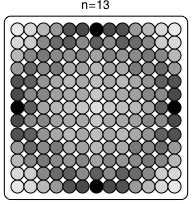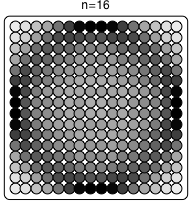Not all grid points are equally likely to appear in solutions to the problem. In general, points near the corners and the center of the grid appear in fewer solutions than points which are on, or slightly inside, the inscribed circle touching the edges of the grid.

The images above illustrate this phenomenon for a few of the largest grid sizes where all solutions are known. Points are colored darker when they appear in more solutions.

This correlates roughly inversely with the number of collinear triples in the grid which contain the point. For example, the corner points occur in a large number of collinear triples, but few solutions. Points near the centers of the edges occur in relatively few collinear triples, and occur in correspondingly more solutions.

Among the known solutions, it's not hard to find individual examples where the markers cluster around the circle, and avoid the corners and center. There are some examples on the following pages.

### Very Circular Solutions 1

Just for fun, here are a few selected solutions on medium-sized grids which show a tendency to cluster near the circle.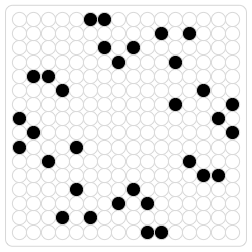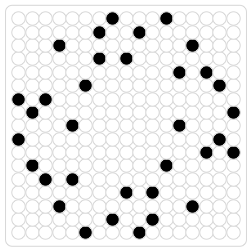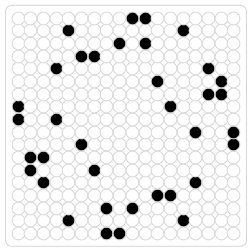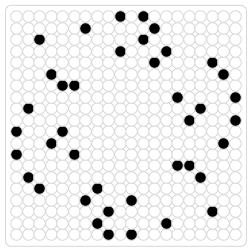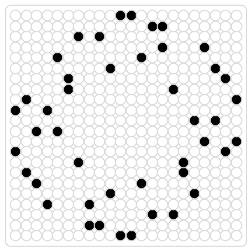### Very Circular Solutions 2

...and here are a few of the larger known solutions showing a high degree of circularity (the largest of these is a 36×36 grid).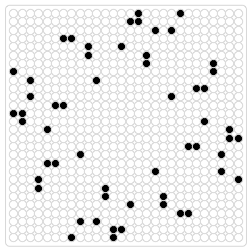### Things You Could Do

In the following, n refers to the size of the grid, and "solution" means a configuration of 2n markers on the n×n grid with no three in line. None of these would be trivial, and some of them are almost certain to be ferociously hard, but at least some of them are feasible and potentially rewarding.

1. Find some new solutions which aren't already known. What is and isn't known:
• All the solutions up to n=16 are already known, and available from Flammenkamp's site. Someone probably knows the solutions for n=17 and n=18, but they aren't published or accessible as far as I know.
• For n=19 up to n=46, some (but not all) solutions are known. Those which are known have special symmetries. Any asymmetric solution with n>18 would be a new discovery.
• There is only a single solution known for each of n=48, 50, and 52. These all have four-fold rotational symmetry. There are probably many others to be discovered.
• There are no solutions known for n=47, 49, or 51. These odd sizes don't permit as many symmetries, making it harder to search for solutions.
• No solutions are known for any n>52.
2. Find a general construction which can be used to place a large number of markers on the n×n grid with no three in line, even if it achieves less than 2n. A construction which achieves more than (3n/2) would be new. Existing constructions (see the references below) use the idea of identifying the n×n grid with ordered pairs of integers modulo n, and using pairs that satisfy some quadratic equation(s).
3. Prove or disprove: there are no solutions larger than 10x10 with full symmetry.
4. Prove or disprove: Every solution that has horizontal and vertical lines of symmetry has full symmetry (including 4-fold rotation).
5. Find some (large) value of n for which you can prove that there is no solution (i.e., the maximum number of markers with no three in line is less than 2n)
6. Prove that, for all sufficiently large n, there are no solutions. Or defy expectations and prove that there are solutions of arbitrarily large sizes.
7. Find the largest value of n for which a solution exists (if there is such a largest value).

Most of these references have a further list of references of their own. I've tried to select a few of the most useful starting points.

1. No Three in Line Problem (Achim Flammenkamp)

Although it hasn't been updated much in many years, Flammenkamp's page is still the most thorough clearinghouse for information solutions to the problem. There's not much theory there.

2. Sequence A00769 in the Online Encyclopedia of Integer Sequences

Gives the number of inequivalent solutions on the n×n grid (up to n=18, currently). Also has a list of references relevant to this problem and some of its close relatives.

3. Random no-three-in-line sets (David Eppstein)

Includes some more current discussion of the asymptotic problem (when n is large, how many markers should we expect to be able to place). Also, why it may be optimistic to expect that we will find the best (largest possible) solutions on large grids by algebraic constructions of the type found in Hall, Jackson, et al.

4. The No-Three-in-Line Problem (Richard K. Guy and Patrick Kelly, 1968)

This is the source for a number of the conjectures mentioned above, and includes an early discussion of the asymptotic problem. A much shorter version of this was published in the Canadian Mathematical Bulletin; the version I have linked includes more detail and interesting discussion.

5. Some advances in the no-three-in-line problem (Hall, Jackson, Sudbury and Wild, 1975)

Gives algebraic constructions for placing markers with no three in line. A simple construction places n markers on the n×n grid, and a slightly more complicated one achieves approximately (3/2)n markers when n is large.

6. Back From the Klondike and Other Problems (from Gardner, Penrose Tiles to Trapdoor Ciphers, 1989)

The book has been reissued a few times, but I think this chapter is unchanged since its appearence in 1989.